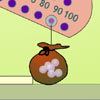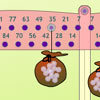#### You may also like### Balancing 1

Meg and Mo need to hang their marbles so that they balance. Use the interactivity to experiment and find out what they need to do.### Balancing 2

Meg and Mo still need to hang their marbles so that they balance, but this time the constraints are different. Use the interactivity to experiment and find out what they need to do.

# Balancing 3

##### Age 11 to 14 Challenge Level:

Well done to Matthias and Aneesh of the Lady Barn House School who made some interesting observations.

If the distance is doubled you need to halve the number of marbles. If the distance is reduced by a factor of 10 you must multiply the number of marbles by 10.
The numbers are also interchangeable so if the distance from the centre is 50cm you will need 16 marbles to balance the scales, and if you are 16cm from the centre you will need 50 marbles to balance the scales.

Congratulations to Jake of Horris Hill who gave a very full answer to this problem.

In his solution, Jake used the term "effective weight" to explain the measure given by weight x distance from the centre, but we have replaced this with the word "moment" which is more conventionally used.

The particular problem with this puzzle is that the bag can be any weight and any distance from the centre, making two factors to consider. However, after experimenting with the scales, I found there was a relation between the weight and distance of one bag and that of the other bag when the scales balanced.

I started to work with the numbers in my head, and I found that the relation was between the products of the two pairs of numbers. In other words: weight of Meg's bag x distance from centre of Meg's bag = weight of Mo's bag x distance from centre of Mo's bag.

Looking at a first class lever (e.g. a see-saw), you can see that the further away the effort (Meg's bag in this case) is from the fulcrum (i.e. middle of the scales), the less force (weight in this case) is needed to lift the load (Mo's bag in this case). This means the bigger the distance and the heavier the bag, the more weight can be lifted, which helps to create a unit of measure that we can compare instead of two separate units.

We call this the moment.

If two bags have the same moment, they will balance. To make it clear what the moment of a bag is, this is the formula:

moment = weight x distance from centre.

Going back to the problem, the unit of the moment is marbles (weight) x cm (distance from middle of scales), and this arms us with a solution to the problem. A quick glance at Mo's bag tells us that the moment is 800 (marbles x cm), meaning that any bag with a moment of 800 (marbles x cm) will balance with it. Once you know this, all you need is one of the measurements

 Distance from the Centre Number of Marbles 100cm 8 50cm 16 25cm 32 40cm 20

 Distance from the Centre Number of Marbles 200cm 4 20cm 40 32cm 25 x 800/x 800/x x

Incidentally, the highest number of marbles possible on the interactivity is 32, meaning the nearest possible distance to the centre whilst keeping the bags balanced is 25 cm (see chart, above).